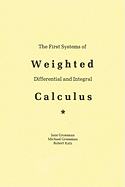## The First Systems Of Weighted Differential And Integral Calculus

This book explains how each non-Newtonian calculus, as well as the classical calculus of Newton and Leibniz, can be 'weighted' in a natural way. In each of these weighted calculi, a weighted average (of functions) plays a central role.The weighted calculi provide a wide variety of mathematical tools for use in science, engineering, and mathematics. They appear to have considerable potential for use as alternatives to the classical calculus. It may well be that they can be used to define new concepts, to yield new or simpler laws, or to formulate or solve problems.

• Condition: New
• Format: Paperback
• Sold by: HPB.com
• Seller rating:
• Language: English
• Publisher: Michael Grossman
• ISBN-13: 9780977117017
• ISBN: 0977117014
• Publication Year: 2006